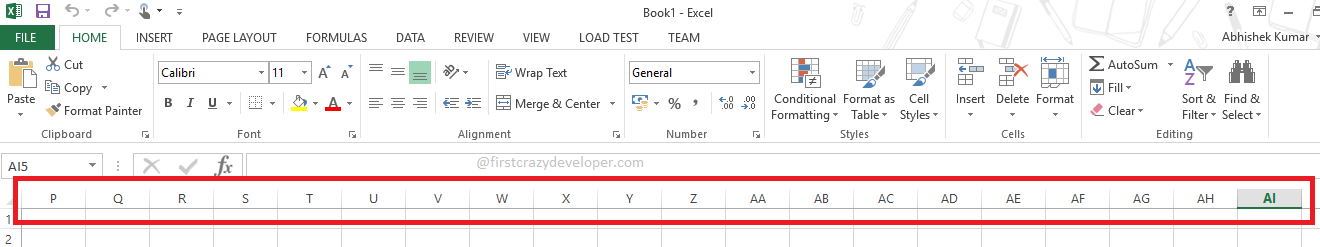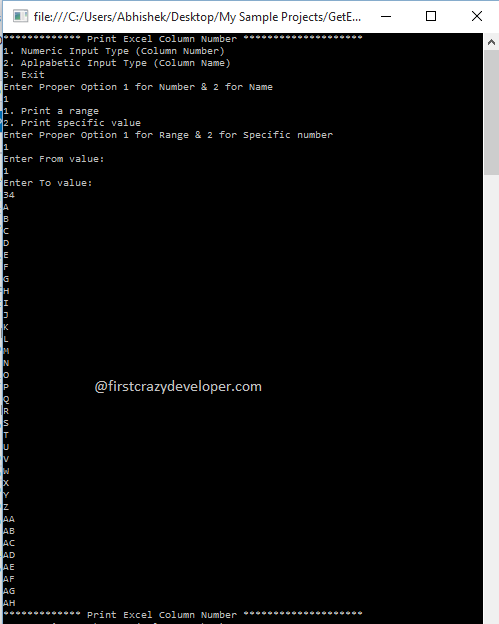© 2021 - First Crazy Developer (Abhishek Kumar)

###Get Excel Column Name & Number in C#

Hi friends, few days back someone asked me a question and that time I misunderstood the question means I didn't understand what they want to ask me? Now I thought I should share that with all of you with proper answer.

Let start with question- “How can we print the Column name of Excel Sheet in C#?" Before going to start this discussion first we should look the excel sheet file.

Look at the following image:In the above image we can see excel sheet and their columns name. Means format of columns name are "A to XFD' and maximum number of column is 16384. That time I was confuse the limit of excel columns. Finally when I came back to home again tried to write the code then after I understood the exact meaning of question.

Now move ahead and we try to understand the above concepts. First we will convert the specific column number into name.

Look at the following code:

1. static string ConvertExcelColumnNumberToName(int columnNumber)
2. {
3. if (columnNumber == null) throw new ArgumentNullException("columnNumber");
4. string setColumnName = String.Empty;
5. int tempRemainder = 0;
6. while (columnNumber > 0)
7. {
8. tempRemainder = (columnNumber - 1) % 26;
9. setColumnName = Convert.ToChar(65 + tempRemainder).ToString() + setColumnName;
10. columnNumber = (int)((columnNumber - tempRemainder) / 26);
11. }
12. return setColumnName;
13. }

In the above code we can identify the logic to convert the number into name. In first line we divided the number with 26 and then get the remainder value. Then after we got the column name and again in next line we set new columnNumber value.

After the above discussion we will get the column number from column name.

Look at the following code:

1. static int ConvertExcelColumnNameToNumber(string columnName)
2. {
3. if (string.IsNullOrEmpty(columnName)) throw new ArgumentNullException("columnName");
4. //Convert Column Name into Upper Case
5. columnName = columnName.ToUpperInvariant();
6. int NumberCalci = 0;
7. for (int i = 0; i < columnName.Length; i++)
8. {
9. NumberCalci = NumberCalci * 26;
10. NumberCalci = NumberCalci + (columnName[i] - 'A' + 1);
11. }
12. return NumberCalci;
13. }

In the above code we can identify the logic to convert the name into number. First we changed the case of column name then after we started looping till column name length. Then after we converted name into number.

After the above code we understood the basic concepts about get the excel column value in C# code. Now move ahead and look the complete code where we can print the series of excel column value.

Look at the following code:

1. using System;
2. using System.Collections.Generic;
3. using System.Linq;
4. using System.Text;
5. using System.Threading.Tasks;
6. namespace GetExcelColumnValues
7. {
8. class PrintExcelColumnValue
9. {
10. static void Main(string[] args)
11. {
12. PrintExcelColumn();
13. }
14. static void PrintExcelColumn()
15. {
16. Console.WriteLine("************* Print Excel Column Number ********************");
17. Console.WriteLine("1. Numeric Input Type (Column Number)");
18. Console.WriteLine("2. Aplpabetic Input Type (Column Name)");
19. Console.WriteLine("3. Exit");
20. Console.WriteLine("Enter Proper Option 1 for Number & 2 for Name");
21. int inputType = 0;
22. int printType = 0;
23. try
24. {
25. inputType = Convert.ToInt32(Console.ReadLine());
26. if (inputType == 3)
27. Environment.Exit(1);
28. Console.WriteLine("1. Print a range");
29. Console.WriteLine("2. Print specific value");
30. Console.WriteLine("Enter Proper Option 1 for Range & 2 for Specific number");
31. printType = Convert.ToInt32(Console.ReadLine());
32. switch (inputType)
33. {
34. case 1:
35. switch (printType)
36. {
37. case 1:
38. Console.WriteLine("Enter From value:");
39. int from = Convert.ToInt32(Console.ReadLine());
40. Console.WriteLine("Enter To value:");
41. int to = Convert.ToInt32(Console.ReadLine());
42. PrintExcelColumnName(from, to);
43. PrintExcelColumn();
44. return;
45. case 2:
46. Console.WriteLine("Enter Column number:");
47. int value = Convert.ToInt32(Console.ReadLine());
48. string getConvertedValue = ConvertExcelColumnNumberToName(value);
49. Console.WriteLine("Column Name of " + value.ToString() + " is= " + getConvertedValue);
50. PrintExcelColumn();
51. return;
52. }
53. return;
54. case 2:
55. switch (printType)
56. {
57. case 1:
58. Console.WriteLine("Enter From value:");
59. string from = (Console.ReadLine());
60. Console.WriteLine("Enter To value:");
61. string to = (Console.ReadLine());
62. PrintExcelColumnNumber(from, to);
63. PrintExcelColumn();
64. return;
65. case 2:
66. Console.WriteLine("Enter Column Name:");
67. string value = (Console.ReadLine());
68. int getConvertedValue = ConvertExcelColumnNameToNumber(value);
69. Console.WriteLine("Column number of " + value + " is= " + getConvertedValue.ToString());
70. PrintExcelColumn();
71. return;
72. }
73. return;
74. default:
75. Console.WriteLine("Input option in not valid.");
76. Console.ReadLine();
77. return;
78. }
79. }
80. catch (Exception ex)
81. {
82. Console.WriteLine("Input value in not valid.");
83. Console.ReadLine();
84. }
85. }
86. static void PrintExcelColumnName(int from, int to)
87. {
88. for (; from <= to; from++)
89. {
90. Console.WriteLine(ConvertExcelColumnNumberToName(from));
91. }
92. }
93. static void PrintExcelColumnNumber(string from, string to)
94. {
95. int tempFrom = ConvertExcelColumnNameToNumber(from);
96. int tempTo = ConvertExcelColumnNameToNumber(to);
97. PrintExcelColumnName(tempFrom, tempTo);
98. }
99. static int ConvertExcelColumnNameToNumber(string columnName)
100. {
101. if (string.IsNullOrEmpty(columnName)) throw new ArgumentNullException("columnName");
102. //Convert Column Name into Upper Case
103. columnName = columnName.ToUpperInvariant();
104. int NumberCalci = 0;
105. for (int i = 0; i < columnName.Length; i++)
106. {
107. NumberCalci = NumberCalci * 26;
108. NumberCalci = NumberCalci + (columnName[i] - 'A' + 1);
109. }
110. return NumberCalci;
111. }
112. static string ConvertExcelColumnNumberToName(int columnNumber)
113. {
114. if (columnNumber == null) throw new ArgumentNullException("columnNumber");
115. string setColumnName = String.Empty;
116. int tempRemainder = 0;
117. while (columnNumber > 0)
118. {
119. tempRemainder = (columnNumber - 1) % 26;
120. setColumnName = Convert.ToChar(65 + tempRemainder).ToString() + setColumnName;
121. columnNumber = (int)((columnNumber - tempRemainder) / 26);
122. }
123. return setColumnName;
124. }
125. }
126. }

In the above code we can see the logic to print the series of column name as well as column number as per user input. When we executed the above code we get the following output.

Look at the following image:I hope we can understood the concepts to print the excel column name and number in C#.

You can find the complete code at: https://github.com/firstcrazydeveloper/GetExcelColumn

Happy reading!!

Abhishek KumarHome Page 07 October 2015